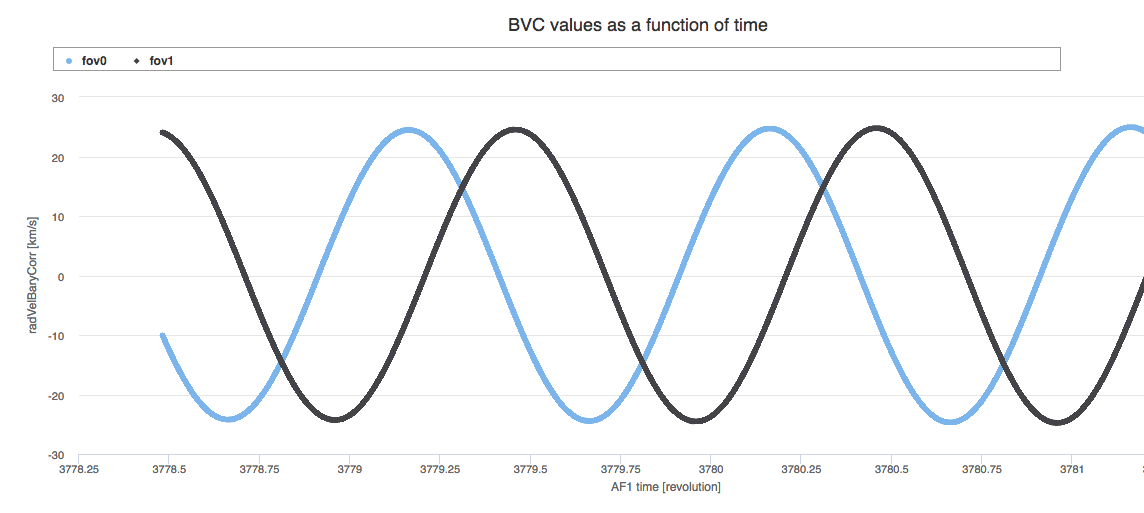6.4.8 Multi-transit analysis

Multi-transit analysis receives single-transit radial velocities and their uncertainties from STA (see Section 6.4.7). The STA Gaia-centric radial velocities are converted to barycentric by adding their barycentric radial velocity correction, which can range from $+30$  km s${}^{-1}$ to $-30$  km s${}^{-1}$ as a function of Gaia’s six hour revolution (Figure 6.6).

The radial velocities $V_{\rm rad}$ published in Gaia DR2 are obtained by taking the median of the STA barycentric single-transit radial velocities $(V_{\rm rad}^{\rm t})$ (Equation 6.1). $V_{\rm rad}$ is computed only if the number of transits $N$ for which a valid $V_{\rm rad}^{\rm t}$ has been obtained is $N\geq 2$ (when $N=2$ the mean value is computed). Not all the transits entering the pipeline result in a $V_{\rm rad}^{\rm t}$. Some transits have been removed from the pipeline upstream STA before calculating $V_{\rm rad}^{\rm t}$ (see Section 6.4.4). The transits flagged as double-lined spectra are also excluded.

The $V_{\rm rad}$ uncertainty provided in Gaia DR2, is given by Equation 6.2, where $N$ is the number of transits used to obtain $V_{\rm rad}$. $N$ is also provided in Gaia DR2.Figure 6.6: Example of barycentric velocity correction as a function of time for the two fields of view for a few OBMT revolutions. The barycentric velocity correction is added to the Gaia-centric single-transit radial velocity computed by STA to obtain the radial velocity with respect to the barycentre of the solar system. The barycentric velocity correction is monitored in the Automated Verification workflow AVPP.